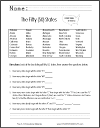Grade 1: Operations and Algebraic Thinking Worksheets
 Share the learning joy!

Grade 1 Math: Operations and Algebraic Thinking
 www.studenthandouts.com > First Grade > First Grade Math > Operations and Algebraic Thinking

CCSS.MATH.CONTENT.1.OA.A.1 - Use addition and subtraction within 20 to solve word problems involving situations of adding to, taking from, putting together, taking apart, and comparing, with unknowns in all positions, e.g., by using objects, drawings, and equations with a symbol for the unknown number to represent the problem.States Math Worksheet
CCSS.MATH.CONTENT.1.OA.A.2 - Solve word problems that call for addition of three whole numbers whose sum is less than or equal to 20, e.g., by using objects, drawings, and equations with a symbol for the unknown number to represent the problem.

CCSS.MATH.CONTENT.1.OA.B.3 - Apply properties of operations as strategies to add and subtract.2 Examples: If 8 + 3 = 11 is known, then 3 + 8 = 11 is also known. (Commutative property of addition.) To add 2 + 6 + 4, the second two numbers can be added to make a ten, so 2 + 6 + 4 = 2 + 10 = 12. (Associative property of addition.)

CCSS.MATH.CONTENT.1.OA.B.4 - Understand subtraction as an unknown-addend problem. For example, subtract 10 - 8 by finding the number that makes 10 when added to 8.

CCSS.MATH.CONTENT.1.OA.C.5 - Relate counting to addition and subtraction (e.g., by counting on 2 to add 2).

CCSS.MATH.CONTENT.1.OA.C.6 - Add and subtract within 20, demonstrating fluency for addition and subtraction within 10. Use strategies such as counting on; making ten (e.g., 8 + 6 = 8 + 2 + 4 = 10 + 4 = 14); decomposing a number leading to a ten (e.g., 13 - 4 = 13 - 3 - 1 = 10 - 1 = 9); using the relationship between addition and subtraction (e.g., knowing that 8 + 4 = 12, one knows 12 - 8 = 4); and creating equivalent but easier or known sums (e.g., adding 6 + 7 by creating the known equivalent 6 + 6 + 1 = 12 + 1 = 13).

CCSS.MATH.CONTENT.1.OA.D.7 - Understand the meaning of the equal sign, and determine if equations involving addition and subtraction are true or false. For example, which of the following equations are true and which are false? 6 = 6, 7 = 8 - 1, 5 + 2 = 2 + 5, 4 + 1 = 5 + 2.

CCSS.MATH.CONTENT.1.OA.D.8 - Determine the unknown whole number in an addition or subtraction equation relating three whole numbers. For example, determine the unknown number that makes the equation true in each of the equations 8 + ? = 11, 5 = _ - 3, 6 + 6 = _.